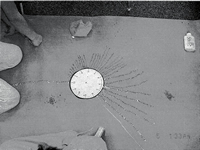# Time Activities

## Exploring a Clock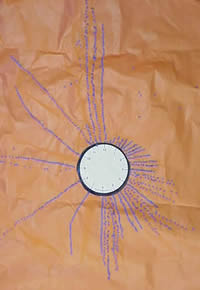1. Start with a very big handless clock with numerals 1-12 (make from a large sheet of craft paper) and put blocks around the perimeter, one for each minute or glue dots on to represent minutes.
2. Count the blocks and write how many are at each point.
3. How many blocks are there at each number (1-12)?
4. What does that mean.
5. Stack blocks to represent the minute value for each numbered place 1-12 (five blocks at the one for five minutes, ten blocks at the two for ten minutes, and so on).
6. Count by fives.
7. How does that compare to counting by ones?
8. Practice counting and have a student point to different numbers and ask the other students what the value is.
9. Discuss strategies to remember what value is at different points around the clock.
10. If desirable put blocks for the other minutes or ask students how many blocks would be at each minute and write the values on the clock.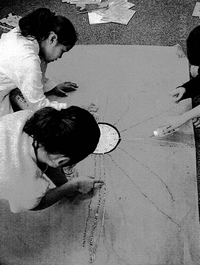Move to a one Handed Clock

1. Next put an hour hand on a clock to make a one handed clock.
2. Move the hand and have students tell the time by saying something like one o'clock, one something, 12 something, or two something…
3. Create a rule that explains how they decide what to say.

### Last a two Handed Clock

1. Introduce a clock with two hands.
2. Have students explain how the hands move
3. What is the relation of each.
4. Have them demonstrate how each hand moves for minutes, hours, and a day.
5. Ask them to write a rule for telling time to the minute and hour.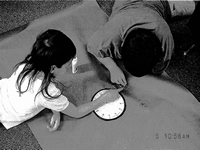Sample rules created by students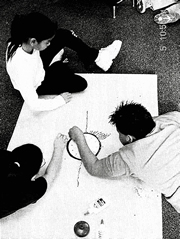### Estimating and Calculating Length of Time

1. Have students show the placement of hands for before, later, earlier…
2. Have students shade the area swept by the hands to tell the time elapsed from one time to another.
3. If they sweep past the hour have them cross off the present hour and continue to do so every time the hand passes another hour.